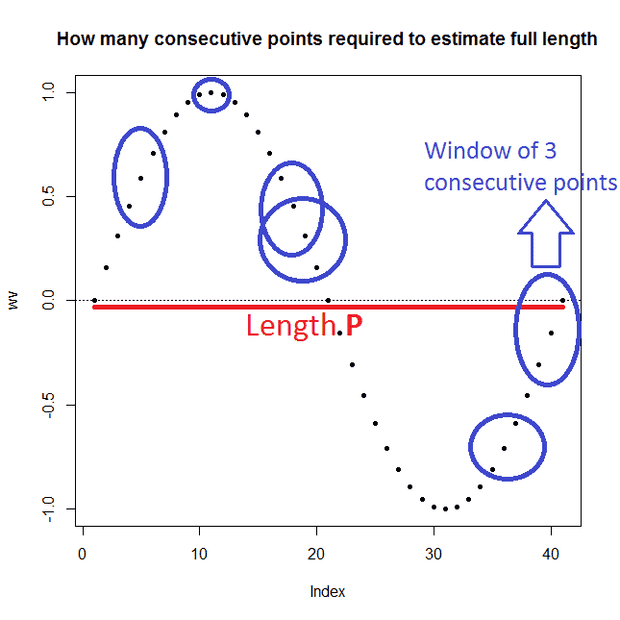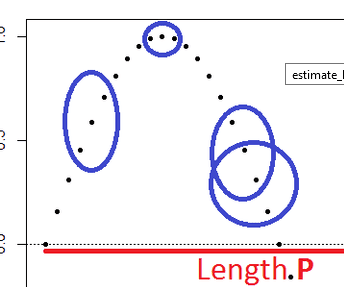# Minimum sample length to estimate the frequency of a sinusoid

• A
MisterH
TL;DR Summary
minimum window length to find the full length of a discretely sampled sinusoid?
Given a discretely sampled horizontal sinusoid of length p, and unknown amplitude, what is the minimal number of consecutive points on a window that is required to correctly estimate its total length, starting at any random point on the wave? Initially I would think it would be either p (full length), or p/2 (half length), right?What if you found a method to correctly estimate its length p on only 3 sample points: would there be a commercial application for this?

Mentor
Are you guaranteed that it is a noise-free pure sinusoid?

MisterH
Yes

Mentor
Then I would think it would be a pretty small number. Is there a reason that you have groups of 3 points in a row circled in the above plot?

Summary:: minimum window length to find the full length of a discretely sampled sinusoid?

What if you found a method to correctly estimate its length p on only 3 sample points: would there be a commercial application for this?
The problem is that commercial applications involve real-world signals that are not pure sinusoids, and generally also involve some level of noise...

MisterH
"Then I would think it would be a pretty small number. Is there a reason that you have groups of 3 points in a row circled in the above plot?"
-> just to illustrate that 1) it can be at any random point and 2) 3 is enough for my method.

"The problem is that commercial applications involve real-world signals that are not pure sinusoids, and generally also involve some level of noise..."
-> Indeed... But given the fact that the sinusoid is more or less the basic shape of nature, I'd think there's something I didn't think of, maybe related to electricity, or signal processing, etc., etc. I'm obviously not a physicist.

Gold Member
When you sample a signal, don't you have to sample at a freq twice the original? That would be a half wavelength which is what you originally thought.

MisterH
"When you sample a signal, don't you have to sample at a freq twice the original? That would be a half wavelength which is what you originally thought."

-> If I understand it correctly, that's "Nyquist frequency", yes.

Mentor
When you sample a signal, don't you have to sample at a freq twice the original? That would be a half wavelength which is what you originally thought.
That's a different situation from what the OP asked originally, if I understood his question and graph:
Summary:: minimum window length to find the full length of a discretely sampled sinusoid?

Given a discretely sampled horizontal sinusoid of length p, and unknown amplitude, what is the minimal number of consecutive points on a window that is required to correctly estimate its total length, starting at any random point on the wave?
It seemed to me that he was implying that the sampling rate was plenty high, he just wanted to be able tell everything about the sinusoid from just a few samples (like not waiting for a complete sinusoid to have to be sampled.

Homework Helper
Gold Member
It depends on how much accuracy you require. The simple Nyquist frequency allows you to get complete accuracy from an infinite sample over an infinite time. Any finite time for the sample will not guarantee complete accuracy. The problem is that you are not guaranteed to get any sample at the exact zero of the sinusoidal. Anything else will give you a possible error for any finite sample.

Mentor
There are only three degrees of freedom - amplitude, frequency and phase. From a purely mathematical point three samples are sufficient if you are guaranteed to sample at a sufficiently high rate with no errors. That's not a real-life situation of course, where you need to consider measurement uncertainties, deviations from a pure sine wave and so on. In that case longer measurement windows will lead to smaller uncertainties.

•jasonRF, pbuk and berkeman
Homework Helper
Gold Member
When you sample a signal, don't you have to sample at a freq twice the original? That would be a half wavelength which is what you originally thought.
In each case the samples indicated in the graphic are ~10x~ the frequency of the original so this is not relevant.
There are only three degrees of freedom - amplitude, frequency and phase. From a purely mathematical point three samples are sufficient if you are guaranteed to sample at a sufficiently high rate with no errors. That's not a real-life situation of course, where you need to consider measurement uncertainties, deviations from a pure sine wave and so on. In that case longer measurement windows will lead to smaller uncertainties.
Hmmm, I think we need more information: the three points in the second set in the graphic could have come from a waveform at ~10x the frequency.

Mentor
Hmmm, I think we need more information: the three points in the second set in the graphic could have come from a waveform at ~10x the frequency.
I don't see that. How so?Homework Helper
Gold Member
First point (in the second group) at ## \frac{\pi}2 \phi - \epsilon ## second at ## 3 \frac{\pi}2 \phi ## third at ## 5 \frac{\pi}2 \phi + \epsilon ##?

Mentor
That's the sampling at a sufficient rate I mentioned. We should be sure the period isn't smaller than our sampling distances.

I don't know if irrational ratios of sampling distances can break that ambiguity already, removing that restriction. What if we sample at times 0,a,b, with a/b irrational?

Homework Helper
Gold Member
Putting aside the practical point that all floating point representations are rational (we are talking about digital sampling?), given that any irrational number can be approximated arbitrarily closely by a rational number I don't see how that could help even in theory: how would the processing engine (whether digital or analogue) tell the difference?

There are only three degrees of freedom - amplitude, frequency and phase. From a purely mathematical point three samples are sufficient if you are guaranteed to sample at a sufficiently high rate with no errors.
If I asked you to interpolate between three equally spaced points in time, using a sine curve, then how might you fit it ?
Note that if the points were co-linear; +v, 0 and -v, then it could not identify the frequency as the data is centred on a zero crossing.

•pbuk
Mentor
Putting aside the practical point that all floating point representations are rational (we are talking about digital sampling?), given that any irrational number can be approximated arbitrarily closely by a rational number I don't see how that could help even in theory: how would the processing engine (whether digital or analogue) tell the difference?
As I said, this is a purely mathematical result. In real life you profit from a larger range.

@Baluncore: Yes, that's a special case where three points are not sufficient. Luckily your chance to hit this case is 0.